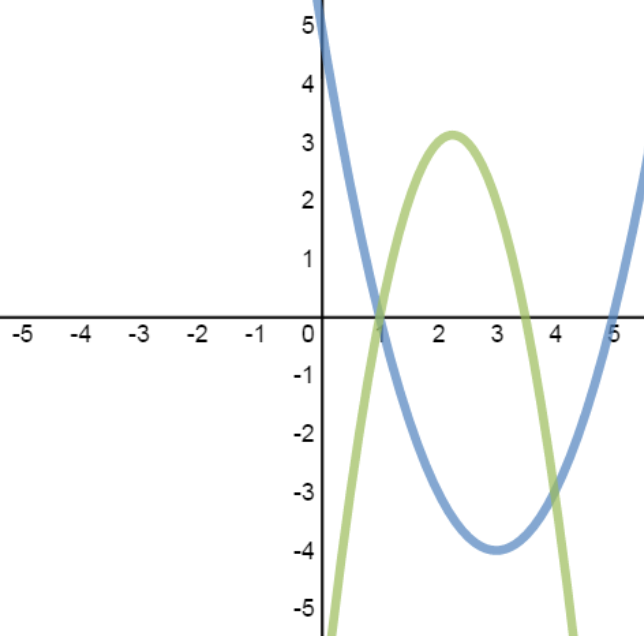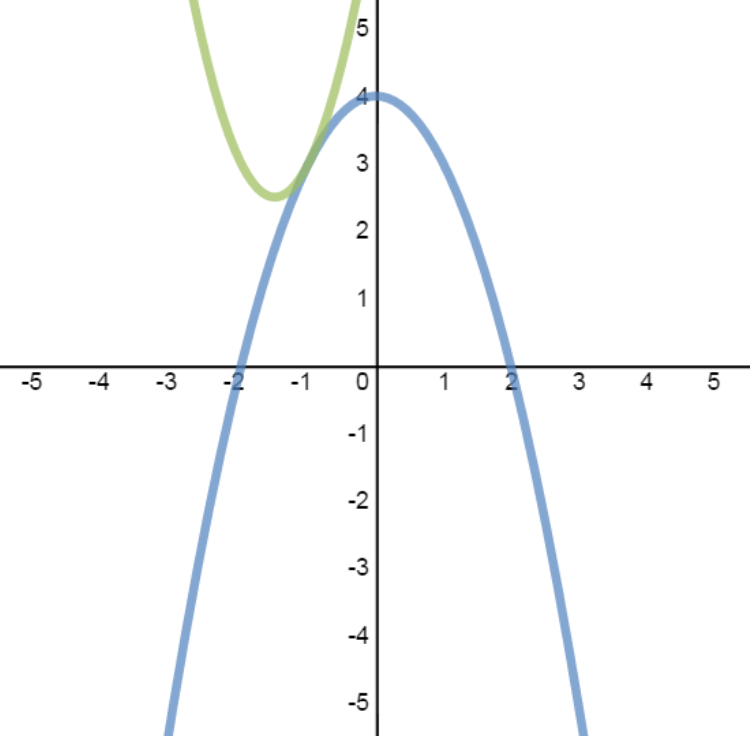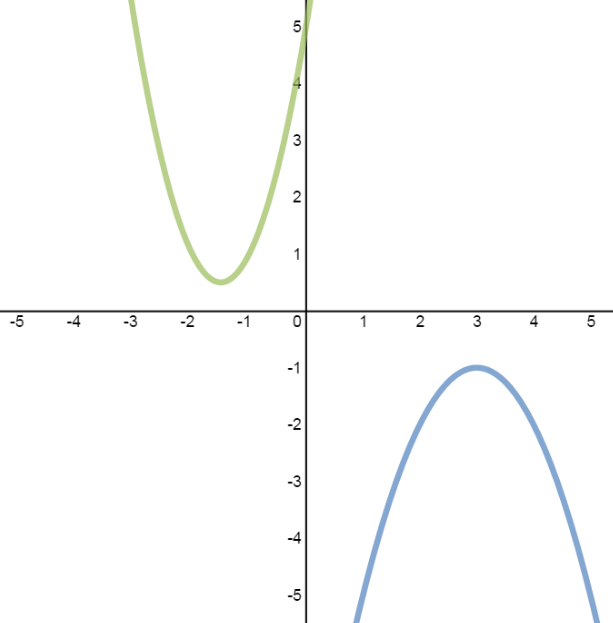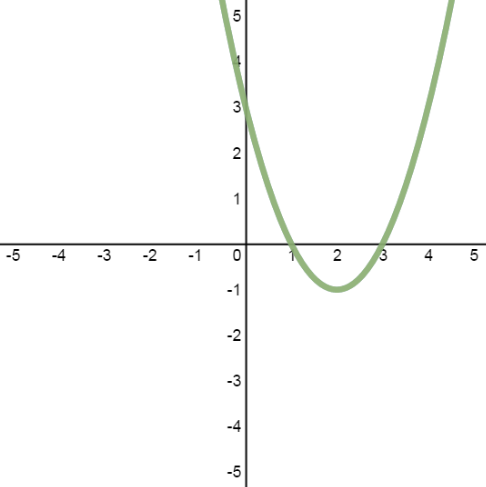#### Everything You Need in One Place

Homework problems? Exam preparation? Trying to grasp a concept or just brushing up the basics? Our extensive help & practice library have got you covered.#### Learn and Practice With Ease

Our proven video lessons ease you through problems quickly, and you get tonnes of friendly practice on questions that trip students up on tests and finals.#### Instant and Unlimited Help

Our personalized learning platform enables you to instantly find the exact walkthrough to your specific type of question. Activate unlimited help now!##### Intros
###### Lessons
1. • The solutions to a system of equations are the points of intersection of the graphs.
• For a system consisting of two quadratic equations:
quadratic equation: $y = a{x^2} + bx + c$
quadratic equation: $y = d{x^2} + ex + f$
There are 4 cases to consider:
case 1: 2 solutions case 2: 1 solution case 3: no solutions case 4: infinite solutions##### Examples
###### Lessons
1. Case 1: System with 2 Solutions
Solve the system, then verify the solutions graphically:
$y = {x^2} - 6x + 5$
$y = - 2{x^2} + 9x - 7$
1. Case 2: System with 1 Solution
Solve the system, then verify the solutions graphically:
$y = 2{x^2} + 6x + 7$
$y = - {x^2} + 4$
1. Case 3: System with No Solutions
Solve the system, then verify the solutions graphically:
$y = - {x^2} + 6x - 10$
$y = 2{x^2} + 6x + 5$
1. Case 4: System with Infinite Solutions
Solve the system, then verify the solutions graphically:
${x^2} - 4x - y + 3 = 0$
$5y - 5{x^2} + 20x - 15 = 0$
###### Topic Notes
The solutions to a system of equations are the points of intersection of the lines. For a system with two quadratic equations, there are 4 cases to consider: 2 solutions, 1 solution, no solutions, and infinite solutions.